# 一、介绍

Embedding-based的模型，比如因子分解机（FM）或深度神经网络，只需要很少的特征工程，通过为每个query和item特征对(pair)学到一个低维的dense embedding vector，可以泛化到之前未见过的query-item特征对，。然而，当底层的query-item矩阵很稀疏和高秩（high-rank）时（比如，用户具有特殊偏好或很少出现的items），很难为query-item pair学到有效的低维表示。在这种情况下，大多数query-item pairs之间是没有交叉的，但dense embeddings会为所有的query-item pairs生成非零的预测，这样会过泛化（over-generalize），并生成不相关的推荐。另一方面，使用交叉特征转换（cross-product features transformations）的线性模型可以使用更少的参数就能记住（memorize）这些“异常规则（exception rules）”。（embedding 优点：泛化，缺点：稀疏时）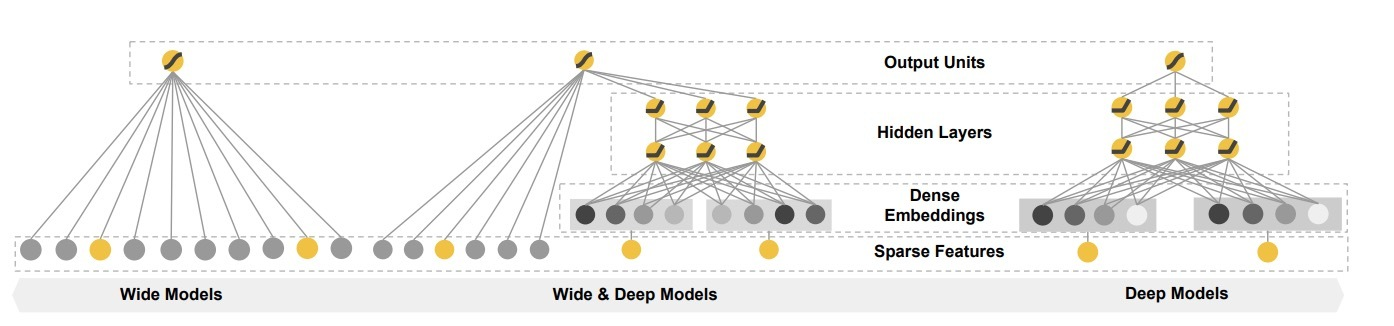• Wide & Deep 学习框架，可以用于联合训练带embeddings的feed-forward神经网络以及带特征转换的线性模型，用于带稀疏输入的常见推荐系统中。
• Wide & Deep推荐系统的实现和评估在Google Play上已经产品化，这个app store具有数十亿的活跃用户、以及上百万的app。
• 开源，在Tensorflow上提供了一个高级API

# 推荐系统总览

app推荐系统如图二所示。一个query，它包含着许多用户和上下文特征，当一个用户访问app store时生成。推荐系统会返回一个app列表（曝光：impressions），用户在此之上会执行特定的动作（点击：click或购买：purchase）。这些用户动作，伴随着queries和impressions，会以日志的形式记录下来。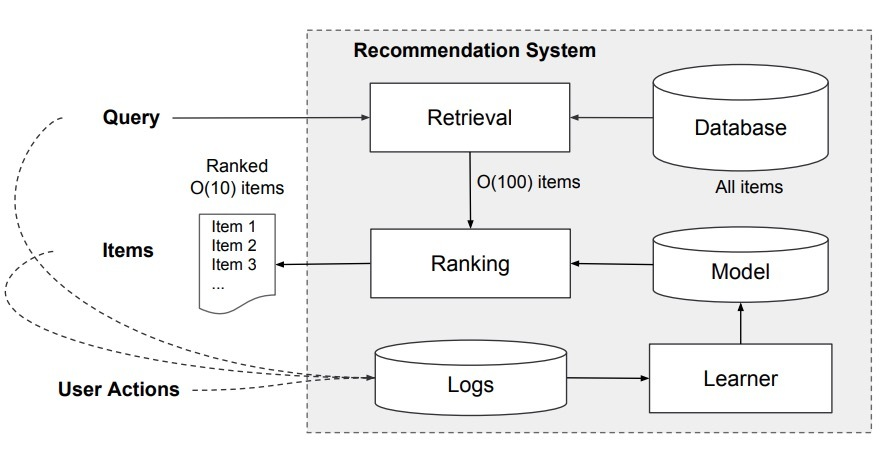# 3. Wide & Deep Learning

## 3.1 Wide组件

wide组件是一个泛化的线性模型，形式为：$y=w^Tx+b$，如图1(左）所示。y是预测，$x = [x_1, x_2, …, x_d]$是d维的特征向量， $w = [w_1, w_2,…, w_d]$是模型参数，其中b为bias。特征集包括原始的输入特征和转换后的特征，一个最重要的转换是，cross-product transformation。它可以定义成：

$\phi_k(x)=\prod_{i=1}^{d}x_{i}^{c_{ki}}, c_{ki} \in \{0, 1\}$

…(1)

## 3.2 Deep组件

Deep组件是一个前馈神经网络(feed-forward NN)，如图1(右）所示。对于类别型特征，原始的输入是特征字符串（比如：”language=en”）。这些稀疏的，高维的类别型特征会首先被转换成一个低维的、dense的、real-valued的向量，通常叫做“embedding vector”。embedding的维度通常是O(10)到O(100)的阶。该embedding vectors被随机初始化，接着最小化最终的loss的方式训练得到该值。这些低维的dense embedding vectors接着通过前向传递被feed给神经网络的隐层。特别地，每个隐层都会执行以下的计算：

$a^{l+1} = f(W^{(l)} a^{(l)} + b^{(l)})$

…(2)

## 3.3 Wide & Deep模型的联合训练

Wide组件和Deep组件组合在一起，对它们的输入日志进行一个加权求和来做为预测，它会被feed给一个常见的logistic loss function来进行联合训练。注意，联合训练（joint training）和集成训练（ensemble）有明显的区别。在ensemble中，每个独立的模型会单独训练，相互并不知道，只有在预测时会组合在一起。相反地，联合训练（joint training）会同时优化所有参数，通过将wide组件和deep组件在训练时进行加权求和的方式进行。这也暗示了模型的size：对于一个ensemble，由于训练是不联合的（disjoint），每个单独的模型size通常需要更大些（例如：更多的特征和转换）来达到合理的精度。相比之下，对于联合训练（joint training）来说，wide组件只需要补充deep组件的缺点，使用一小部分的cross-product特征转换即可，而非使用一个full-size的wide模型

$P(Y = 1 | x) = \sigma(w_{wide}^{T} [x, \phi(x)] + w_{deep}^{T} a^{(l_f)} + b)$

…(3)

# 4.系统实现

app推荐的pipeline实现包含了三个stage：数据生成，模型训练，模型serving。如图3所示。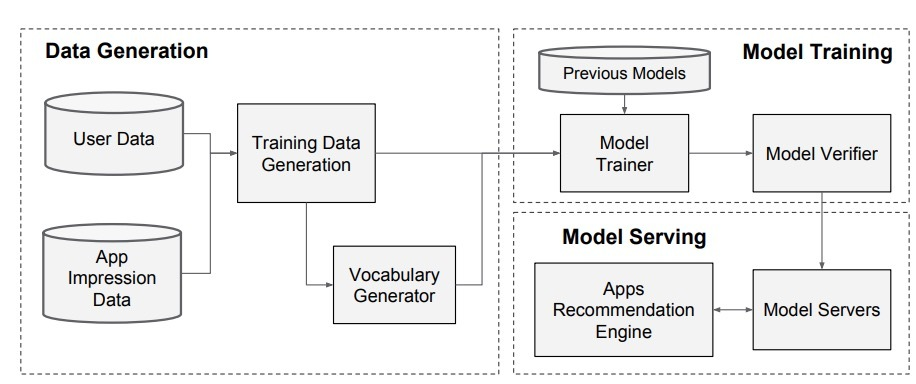## 4.2 模型训练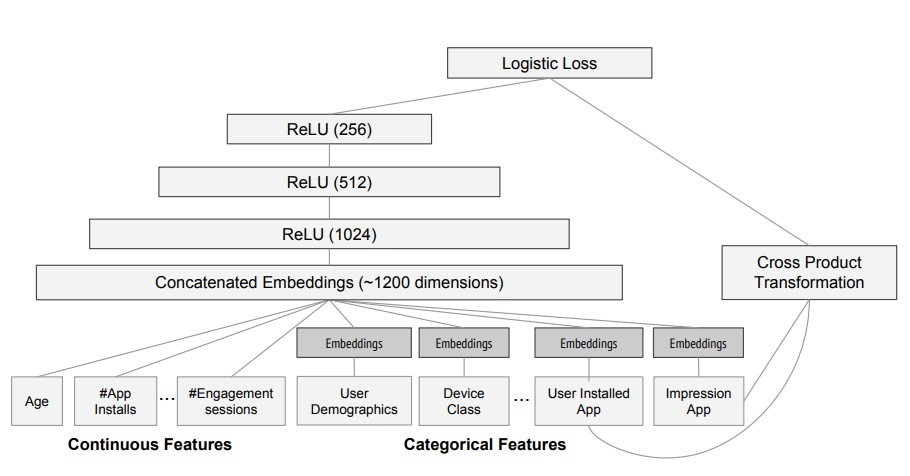Wide & Deep模型在超过5000亿的样本上进行训练。每一时刻有新的训练数据集到达时，模型需要重新训练。然而，每次从头到尾重新训练的计算开销很大，数据到达和模型更新后serving间的延迟较大。为了解决该问题，我们实现了一个warm-starting系统，它会使用前一个模型的embeddings和线性模型权重来初始化一个新的模型

# 5.试验结果

## 5.1 App Acquisitions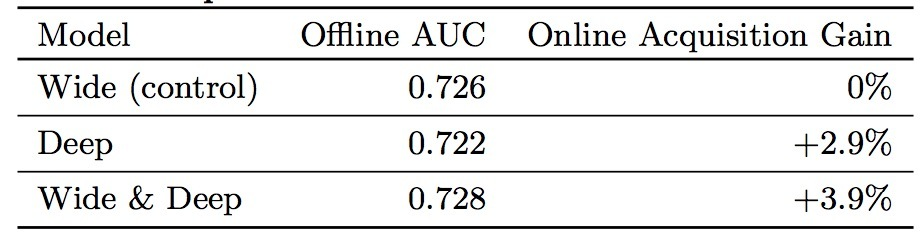## 5.2 Serving Performance

Serving时具有高的吞吐率（throughout）和低延时是很有挑战性的。在高峰期，我们的推荐服务每秒处理1000w个app得分。单个线程上，在单个batch上为所有的候选得进行scoring会花费31ms。我们实现了多线程，并会将每个batch分割到更小的size上，它会将客户端的延迟的延迟减小到14ms上，所图2所示。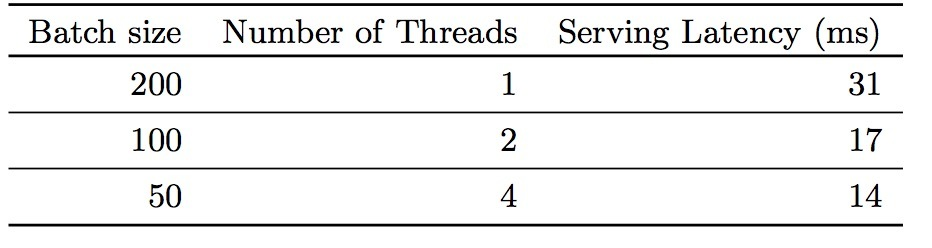# 7.Tensorflow

• 1.选中wide组件的特征：选中你想用的稀疏的base特征列和交叉列特征列
• 2.选择deep组件的特征：选择连续型的列，对于每个类别型列的embedding维，以及隐层的size。
• 将它们放置到一个Wide&Deep模型中（DNNLinearCombinedClassifier）

# 介绍# 2.系统总览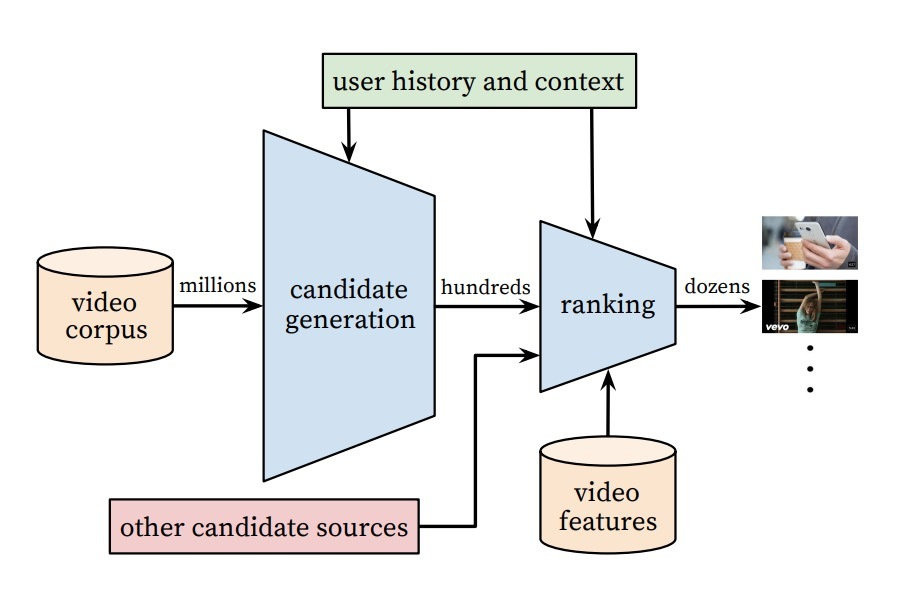# 一、候选生成

## 3.1 推荐即分类

$P(w_t =i|U,C)=\frac{e^{v_{i} u}}{\sum_{j \in V}{e^{v_{j} u}}}$

Efficient Extreme Multiclass

## 1.1 模型架构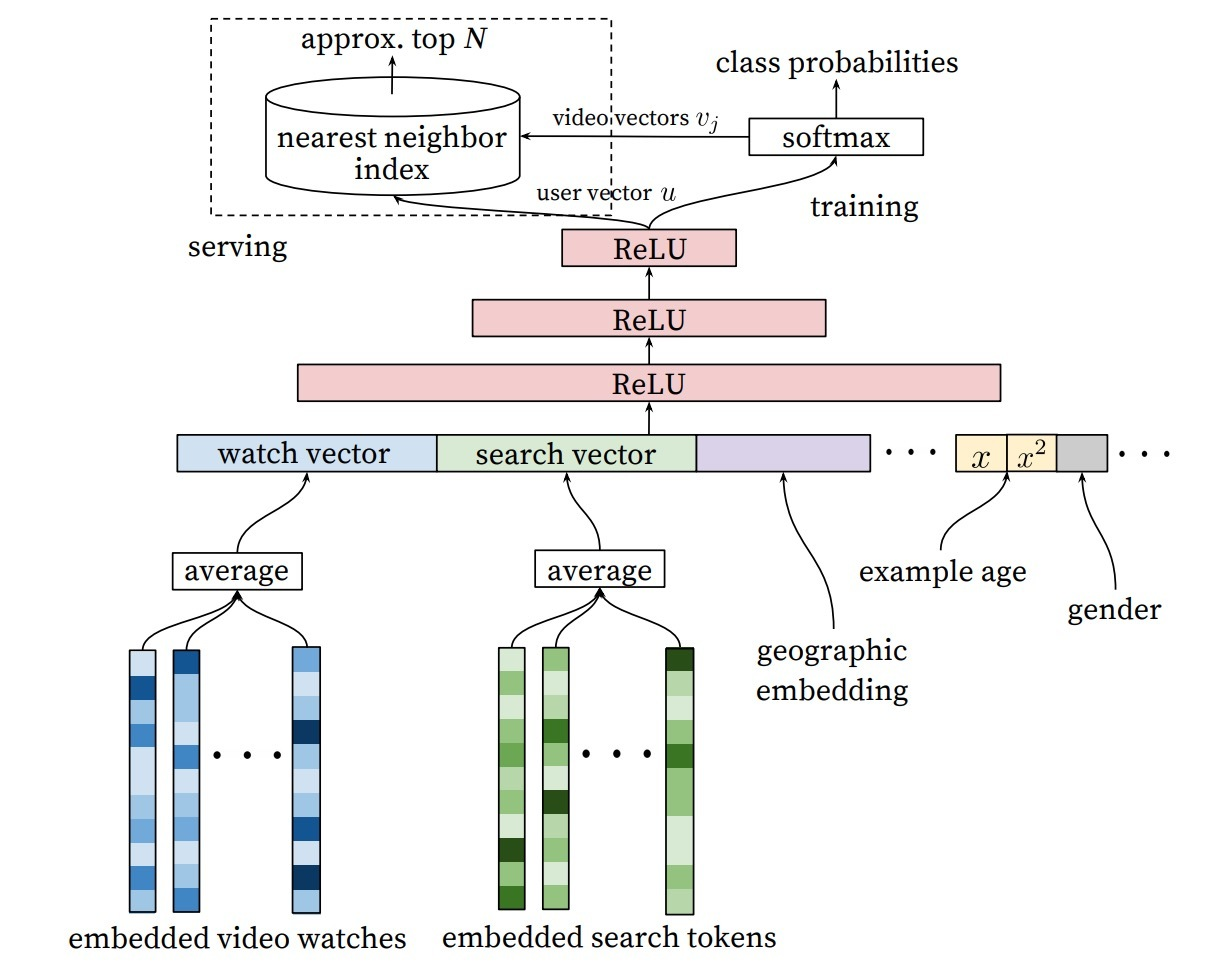## 1.2 多种信号

“样本时限”特征（”Example Age” Feature）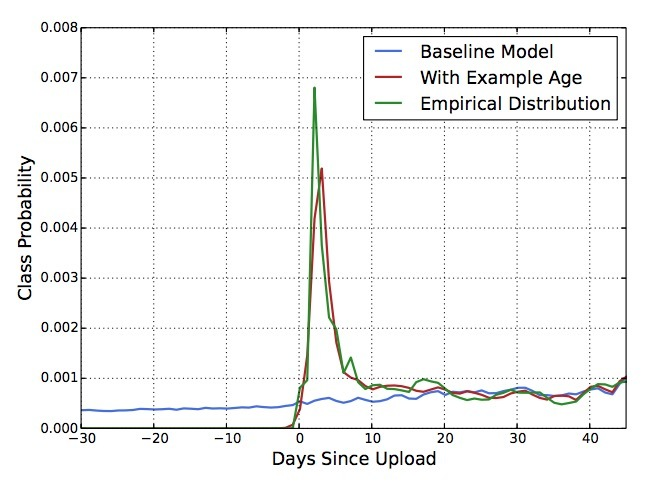## 1.3 Label和Context选择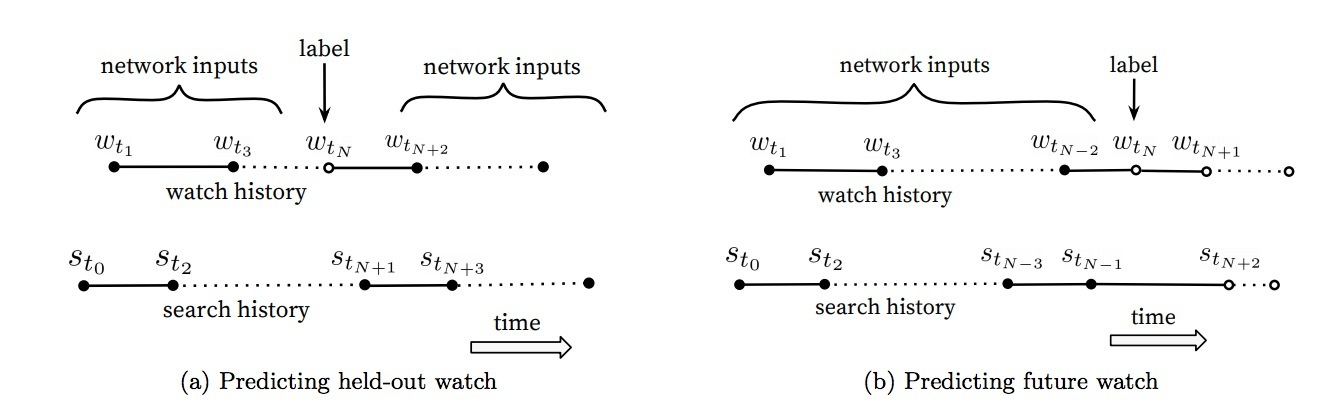## 1.4 特征和深度的试验

• Depth 0: 一个线性层，可简单地将串联层转换成与softmax相匹配的256维.
• Depth 1: 256 ReLU
• Depth 2: 512 ReLU -> 256 ReLU
• Depth 3: 1024 ReLU -> 512 ReLU -> 256 ReLU
• Depth 4: 2048 ReLU -> 1024 ReLU -> 512 ReLU -> 256 ReLU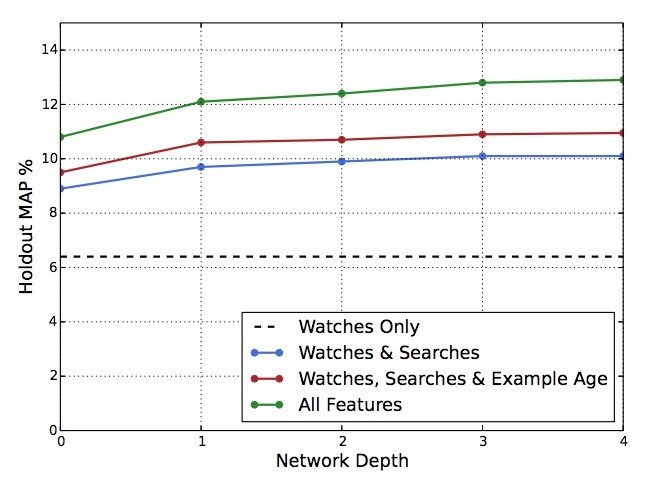# 二、Ranking

Ranking的主要作用是，针对指定的UI，使用曝光数据来特化和校正候选预测（specialized and calibrate candidate predictions）。例如，用户通常会观看一个probability值较高的视频，但不大可能去点击指定主页上缩略图的曝光。在Ranking时，我们会访问许多描述视频的特征、以及视频与用户关系的特征，因为在候选集生成阶段，只有一小部分的视频被打过分，而非上百w的视频。Ranking对于聚合不同的候选源很重要，因为每个源的得分不能直接对比。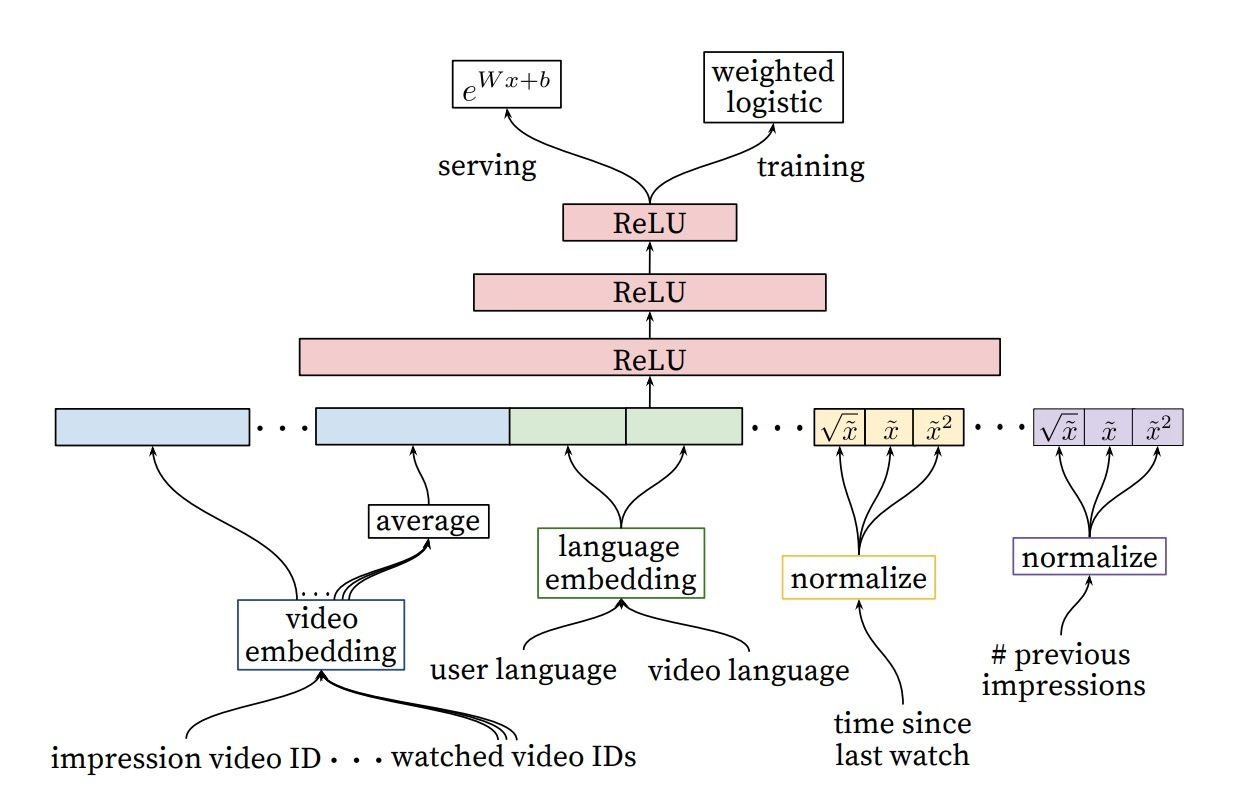## 2.3 隐层的试验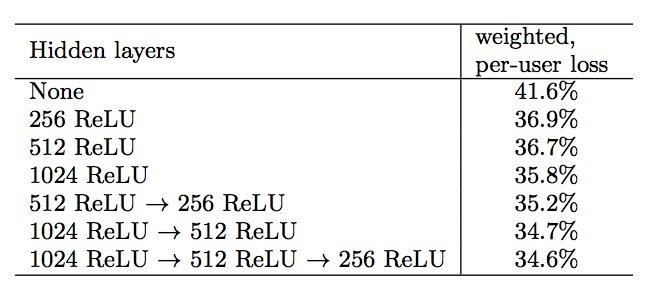# 3.层次化语言模型

## 3.1 模型结构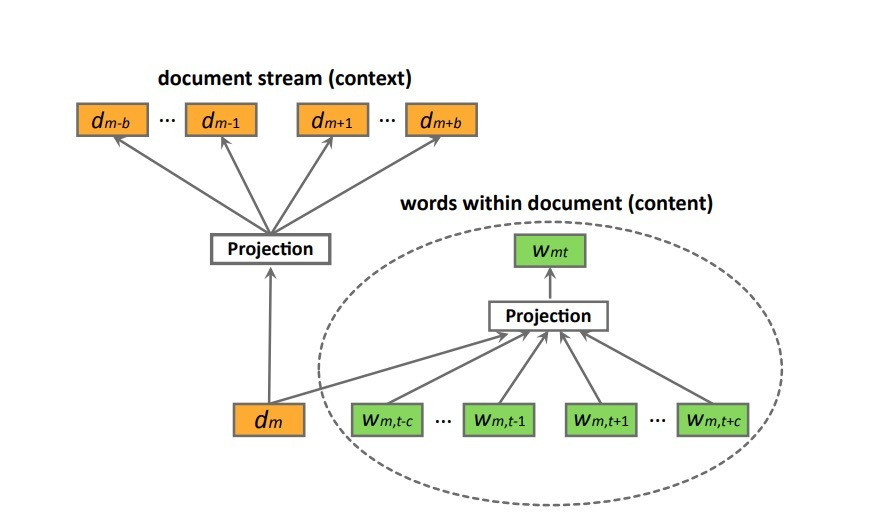$L = \sum_{s \in S} (\alpha \sum_{d_m \in s} \sum_{w_{mt} \in d_m} log P(w_{mt} | w_{m,t-c}: w_{m,t+c}, d) + \\ \sum _{d_m \in s} (\alpha log P(d_m | w_{m1}: w_{mT}) + \sum_{-b \le i \le b, i \neq 0} log P(d_{m+i} | d_m)))$ …(8)

$P(d_{m+i} | d_{m}) = \frac{exp(v_{d_m}^T v_{d_{m+i}}')} {\sum_{d=1}^{M} exp(v_{d_m}^T v_d')}$ …(9)

$P(w_{mt} | w_{m,t-c}: w_{m,t+c}, d_m) = \frac{exp(\bar{v}^T v_{w_{mt}}')} {\sum_{w=1}^{W} exp(\bar{v}^T v_w')}$ …(10)

$\bar{v} = \frac{1}{2c+1} (v_{d_m} + \sum_{-c \leq j \leq c, j \neq 0} v_{w_{m,t+j}})$ …(11)

## 3.3 模型最优化

$P(d_{m+i} \mid d_m) = \prod_l P(h_l \mid q_l, d_m)$ …(12)

$P(h_l = 1 \mid q_l, d_m) = \sigma(v_{d_m}^T V_{q_l})$ …(13)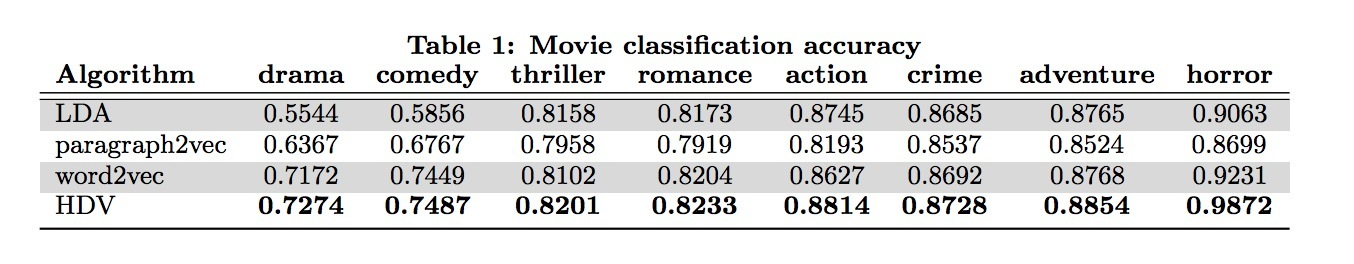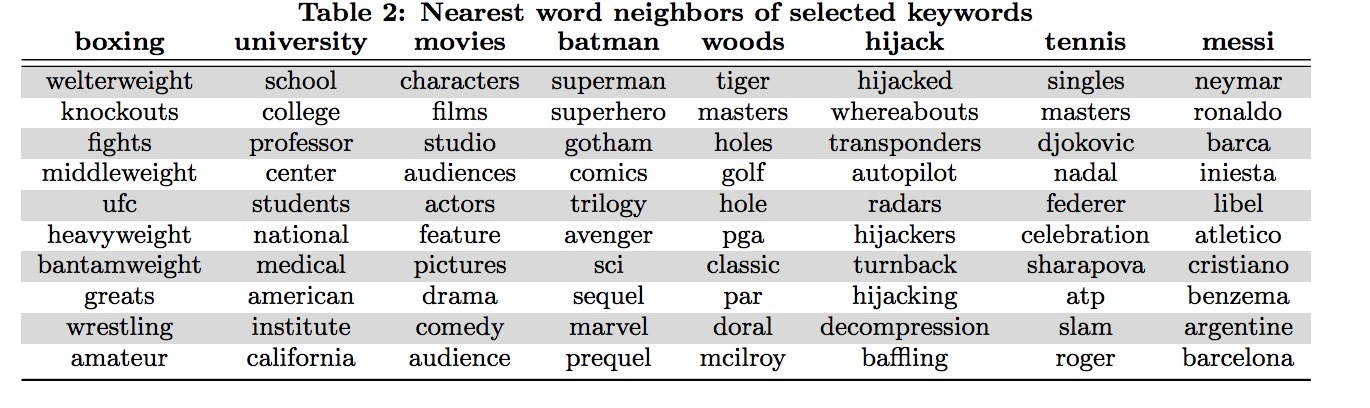# 1.介绍DeepWalk会将一个图作为输入，并生成一个隐表示作为它的输出。将我们的方法应用于Karate network上，结果如图1所示。图1a通过力导向（force-directed）的布局进行表示，图1b展示了我们使用2个隐维度的方法。除了显著的相似性外，我们注意到1b中线性可分的部分，对应于通过输入图1a的模块最大化（modularity maximization）的聚类（clusters）: 通过顶点颜色区分。

DeepWalk比其它用于创建社交维度（social dimension）隐表示方法要好，尤其是当带label的节点（labeled nodes）很稀少时。我们的表示法的性能可能与最简单的分类器（LR）相当。我们的表示是可泛化的，可以结合任何分类方法（包含迭代型推断法）。DeepWalk可以完成上述所有，它是一个在线算法，可并行化。

• 引入深度学习作为一个工具来分析图，来构建健壮的表示，很适合于统计建模。DeepWalk会学到在短随机游走(short random walks)内表示的结构化规律。
• 我们对多个社交网络上使用我们的表示法，并在多标签分类上进行评估。我们发现可以极大提升存在标签稀疏性情况的分类效果，在Micro F1上可以提升5%-10%。在一些case中，即使只给了更少的60%的数据，DeepWalk的表示也可以胜出其它对手。

# 3.学习社交表示

• 社群意识（Community aware）：隐维度间的距离可以作为评估网络成员的社交相似性的一个metric。这允许泛化到同质的网络中。
• 低维（Low dimensional）：当标记数据很少时，低维模型泛化更好，加速收敛和推断。
• 连续（Continuous）：我们需要隐表示来建模在连续空间中的部分社群成员关系（community membership）。除了提供一个关于社群关系的细微视图外，连续表示法具有在社群间的平滑决策边界，这可以允许更健壮的分类。

## 3.1 随机游走

• 1.局部探索（local exploration）很容易并行化。多个随机游走者（random walkers）以不同线程、进程、机器可以并行探索同一个graph下的不同部分。
• 2.依靠从短随机游走上获取信息，可以使它更能适应在图结构上的小变动，无需进行全局重新计算。我们可以迭代式地更新学到的模型，从变动区域上使用新的随机游走，它对整个graph是在时间上是次线性的。

## 3.2 连接：二八法则（power law）## 3.3 语言建模

$W_1^n = (w_0, w_1, ..., w_n)$

$Pr(v_i | (v_1, v_2, ..., v_{i-1}))$

$Pr(v_i | (\Phi(v_1), \Phi(v_2), ..., \Phi(v_{i-1})))$ …(1)

$minimize_{\Phi} - log Pr({v_{i-w}, ..., v_{i-1}, v_{i+1}, ..., v_{i+w}} | \Phi(v_i))$ …(2)

# 4.方法

## 4.2 算法：DeepWalk

• 1.随机游走生成器
• 2.一个更新过程### 4.2.1 SkipGram

SkipGram是一个语言模型，在一个句子中，它会最大化在同一窗口w中词之间的共现率。### 4.2.2 Hierarchical Softmax

$Pr(u_k | \Phi(v_j)) = \prod_{i=1}^{log|V|} Pr(b_l | \Phi(v_j))$

## 4.3 并行化(Parallelizability)# 参考

DeepWalk: Online Learning of Social Representations

# 1.介绍# 2.方法

## 2.1 引入skip-ghought vectors

Encoder：假设$w_i^1, ..., w_i^N$是句子$s_i$中的words，其中N表示在句子中的words数目。在每个step中，encoder会产生一个hidden state：$h_i^t$，它可以解释成序列$w_i^1,...,w_i^t$的表示（representation）。hidden state：$h_i^N$可以表示整个句子。

$r^t = \sigma(W_r x^t + U_r h^{t-1})$ … (1)

$z^t = \sigma(W_z x^t + U_z h^{t-1})$ … (2)

$\bar{h}^t = tanh(W x^t + U (r^t \odot h^{t-1})$ … (3)

$h^t = (1-z^t) \odot h^{t-1} + z^t \odot \bar{h}^t$ …(4)

Decoder: decoder是一个神经语言模型，它的条件是encoder output $h_i$。该计算与encoder相似，除了我们引入了矩阵$C_z，C_r$，以及C，它们被用于偏置由句子向量计算得到的update gate，reset gate和hidden state。一个decoder会被用于下一个句子$s_{i+1}$，而第二个decoder被用于前一句子$s_{i-1}$。Separate参数被用于每个decoder，除了词汇矩阵V，它的权重矩阵会连接decoder的hidden state，以便计算在词上的一个分布。我们在下一个句子$s_{i+1}$上描述decoder，通过一个在前一句子$s_{i-1}$上的类似计算得到。假设$h_{i+1}^t$表示decoder在时间t的hidden state。对下面的等式进行迭代（丢掉下标i+1）：

$r^t = \sigma(W_r^d x^{t-1} + U_r^d h^{t-1} + C_r h_i)$ …(5)

$z^t = \sigma(W_z^d x^{t-1} + U_z^d h^{t-1} + C_z h_i)$ …(6)

$\bar{h}^t = tanh(W^d x^{t-1} + U^d (r^t \odot h^{t-1} + C h_i$ …(7)

$h_{i+1}^t = (1-z^t) \odot h^{t-1} + z^t \odot \bar{h}^t$ …(8)

$P(w_{i+1}^t | w_{i+1}^{<t}, h_i) \propto exp(v_{w_{i+1}^t} h_{i+1}^t )$ …(9)

$\sum_{t} log P(w_{i+1}^t | w_{i+1}^{<t}, h_i) + \sum_{t} log P(w_{i-1}^t | w_{i-1}^{<t}, h_i)$ …(10)

# 3 实验

• 使用学到的encoder作为一个feature extractor，抽取所有句子的skip-thought vectors
• 如果该任务涉及到计算句子对（pairs of sentences）之间的分数，会计算pairs间的component-wise features。
• 在抽取的features上训练一个线性分类器，在skip-thoughts模型中没有任何额外的fine-tuning或者backpropagation。

t-sne.

# 参考

Convolutional Neural Networks for Sentence Classification